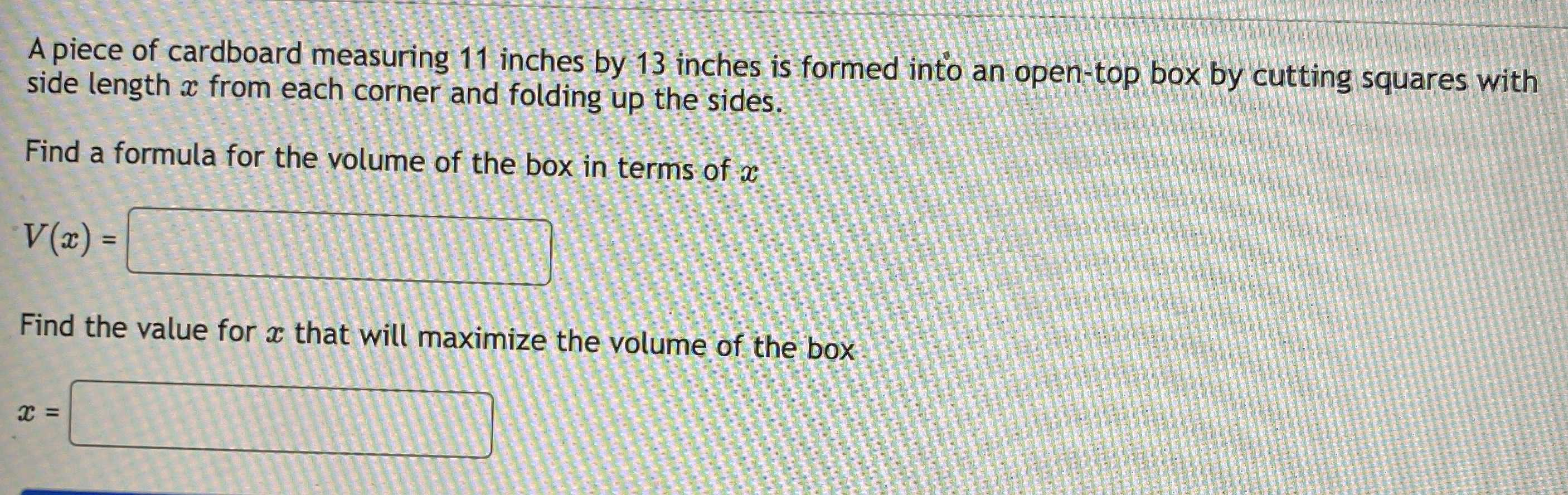### Still have math questions?

Algebra
QuestionA piece of cardboard measuring $$11$$ inches by $$13$$ inches is formed into an open-top box by cutting squares with side length $$x$$ from each corner and folding up the sides. Find a formula for the volume of the box in terms of $$x$$

v(x)=$$x(11- 2x)(13- 2x)$$
x=$$4- \frac{7}{2\sqrt{3} }$$